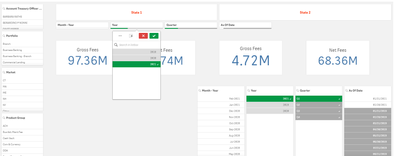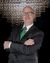# App Development

Announcements
Join “Do More With Qlik” with Mike Tarallo to get a first-hand look at the New Authoring Experience, June 29th: REGISTER HERE
cancel
Showing results for
Did you mean:Contributor III

## Calculating Measures as per Monthly/Quaterly/Yearly selection made on Filter Pane.

Hello, First of all I am a novice, but I have decided to learn Qlik,

For many of you, this sounds simple question, but I have spent enough time on this and I feel that it is time to seek for help.

I am trying to use the filter pane so that
Gross fees is calculated as per date/month/quarter/year -- whatever range user wants to select.For example if I select Year 2021 and Quarter as Q1 I should get the sum of gross for all the months  that falls on Quarter 1 of year 2021.

Currently, the way the app is behaving is, the sum is coming as total cumulative sum (here the sum of  #uvGrossFee), irrespective of the year or quarter I select.

If I select Year 2019 -  I am getting gross as 38.83 M

If I select Year 2020- I am getting gross as 88 M

and If I select 2021 - I am getting 97.36 Million.

Looks like value is getting cumulative - 96M. For  2021, there are only 2 months of data and the value should come around 8 M.

Can someone point me what adjustment I should make on my set analysis?

I tried to calculate the Gross fees as below. Tried some other approaches as well.

sum({<[_Acct_Appl_Code_Flag] ={1},[Cycle End Date]={'>=\$(=MonthStart(Min([Cycle End Date])))'} >} [#uvGross Fee])

or
sum({<_CMTD_Flag ={1},[_Acct_Appl_Code_Flag] ={1} >} [#uvGross Fee])

For reference, I have created a  Master Calendar table as below and I am fetching Month, Quarter, year from this table.

Temp_Dates:
FieldValue('Cycle End Date', RecNo()) as "Cycle End Date"
AutoGenerate
FieldValueCount('Cycle End Date')
;

Join (Temp_Dates)

Date(floor(MonthEnd(AddMonths(MinDate, IterNo() -1)))) as [As Of Date]
WHILE
;

Date(floor(MonthEnd(Min("Cycle End Date")))) as MinDate,
Date(floor(MonthEnd(Max("Cycle End Date")))) as MaxDate
resident
Temp_Dates
;

AsOfDate:
"Cycle End Date",
[As Of Date] ,
Year([As Of Date] ) AS Year,
Month([As Of Date] ) AS Month,
'Q' & ceil(month([As Of Date] ) / 3) AS Quarter,
Year([As Of Date] ) &'-'& 'Q' & ceil(month([As Of Date] ) / 3)
AS QuarterYear,

Date([As Of Date] , 'MMM-YYYY') AS MonthYear,

InMonth("Cycle End Date", [As Of Date] , 0) * -1 AS _CMTD_Flag,
InMonth("Cycle End Date", [As Of Date] , -12) * -1 AS _PMTD_Flag,
InMonth("Cycle End Date", [As Of Date] , -1) * -1 AS _PM_Flag,

InYearToDate("Cycle End Date", [As Of Date] , 0) * -1 AS _CYTD_Flag,
InYearToDate("Cycle End Date", [As Of Date] , -1) * -1 AS _PYTD_Flag,
(AddMonths("Cycle End Date", 12) > [As Of Date] ) * -1 AS _R12_Flag,
(AddMonths("Cycle End Date", 12) <= [As Of Date] ) *
(AddMonths("Cycle End Date", 24) > [As Of Date] ) AS _PR12_Flag,

(AddMonths("Cycle End Date", 6) > [As Of Date] ) * -1 AS _R6_Flag,

(AddMonths("Cycle End Date", 6) <= [As Of Date] ) *
(AddMonths("Cycle End Date", 12) > [As Of Date] ) AS _PR6_Flag,

(AddMonths("Cycle End Date", 12) <= [As Of Date] ) *
(AddMonths("Cycle End Date", 18) > [As Of Date] ) AS _PYR6_Flag,

(AddMonths("Cycle End Date", 3) > [As Of Date] ) * -1 AS _R3_Flag,

(AddMonths("Cycle End Date", 3) <= [As Of Date] ) *
(AddMonths("Cycle End Date", 6) > [As Of Date] ) AS _PR3_Flag,

(AddMonths("Cycle End Date", 12) <= [As Of Date] ) *
(AddMonths("Cycle End Date", 15) > [As Of Date] ) AS _PYR3_Flag

resident
Temp_Dates
where
"Cycle End Date"<=[As Of Date]
;

drop table Temp_Dates;

2 RepliesEmployee

Your Year Month field seems to be messed up. Although you filtered to 2021 it is showing them all.Contributor III
Author

Hi Dalton, That is why I was seeking help.

From further research, what I have found so far is, As of Date solution requires that a single As of Date should be selected at all times.  I am still trying to figure out the solution for this.